# MCAT Physical : General Principles

## Example Questions

← Previous 1 3

### Example Question #1 : Scalar And Vector Quantities

A student measures the mass, volume, weight, and density of a solid in SI units. Which measurement(s) has/have units that are not a type of derived unit?

Mass and weight

Weight and density

Mass and volume

Only mass

Only mass

Explanation:

There are two types of units: base units and derived units. Base units are a set of units from which all other units are derived, whereas derived units are the units derived from base units.

There are six main base units: meters, kilogram, second, Ampere, Kelvin, moles, and candela (unit for luminous intensity). The rest of the units are classified as derived units and will contain a combination of these base units. The SI units for the four measurements listed in question are as follows:

Mass: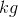Volume: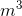(Liters)

Weight: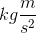(Newtons)

Density: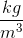This means that volume, weight, and density are derived units, whereas mass is a base unit.

### Example Question #2 : Scalar And Vector Quantities

Which of the following is true about SI derived units and base units?

There are more base units than derived units

Hours are a type of SI base unit

Units for force can be either derived units or base units

Derived units are obtained by adding, subtracting, multiplying, and/or dividing base units

Hours are a type of SI base unit

Explanation:

Derived units are derived from the six base units. A derived unit usually contains multiple base units that are combined by using multiplication and/or division; addition and subtraction of base units is not performed to obtain derived units.

The SI unit for force is Newtons. Newtons written in base units is; therefore, units for force is always a derived unit. A unit can be either a derived unit or a base unit, but it can never be both. Recall that there are only six base units and numerous derived units; therefore, there are more derived units than base units.

The SI base unit for time is seconds. Hours are derived by multiplying seconds by sixty. Hours are not a base unit.

### Example Question #3 : Scalar And Vector Quantities

The unit for mass is a type of __________ unit. The unit "moles" is a type of __________ unit.

base . . . base

base . . . derived

derived . . . derived

derived . . . base

base . . . base

Explanation:

Recall that the mass of a substance is usually measured in kilograms (), which is a type of base unit. Moles (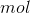) is a unit used to measure the amount of substance. Moles are also a type of base unit; therefore, both the unit for mass () and the unit for amount of substance () are measures of base units.

### Example Question #1 : Linear Motion

Which answer choice below includes only scalar quantities?

Velocity, displacement, force

Acceleration, energy, displacement

Distance, speed, time

Force, time, velocity

Displacement, time, acceleration

Distance, speed, time

Explanation:

Scalar quantities are those that can be described with magnitude only, as opposed to vectors, which include both magnitude and direction components. Distance, speed, and time are all scalars. Displacement is not a scalar, as it involves both the distance and the direction moved from a starting point. Velocity also includes a direction component, and is therefore a vector quantity.

### Example Question #4 : Scalar And Vector Quantities

An airplane is in flight. Which of the following is an example of a vector?

The speed of the plane

The angle at which the plane flies

None of these are vectors

The velocity of the plane

The distance traveled by the plane

The velocity of the plane

Explanation:

A vector quantity has has both magnitude and direction. By definition, only velocity satisfies these criteria.

Distance, angle, and speed have magnitude, but no direction.

### Example Question #1 : Newton's Laws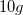ball is rolled into a stationary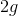ball. If the heavier ball applies a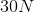force on the smaller ball, what force will the smaller ball apply on the large ball?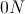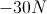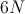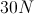Explanation:

The answer to this question lies within Newton's third law: for every action, there is an equal and opposite reaction. If the large ball applies aforce on the smaller ball, the smaller ball will apply aforce on the large ball in the opposite (negative) direction.

### Example Question #2 : Newton's Laws

A 1kg plate falls from a shelf 1m above the ground and breaks into three pieces of mass 0.1kg, 0.4kg and 0.5kg. What is the total energy of the scattered pieces?

We must know the velocity of each piece to solve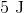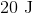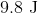We must know the shape of the plate to solveExplanation:

This is a conservation of energy question. The event is the collision. The initial energy can be calculated by gravitational potential energy: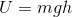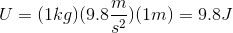The final total energy must be the same. The energy of the scattered pieces must add to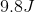### Example Question #1 : Displacement, Velocity, And Acceleration

A 2kg mass is suspended on a rope that wraps around a frictionless pulley attached to the ceiling with a mass of 0.01kg and a radius of 0.25m. The other end of the rope is attached to a massless suspended platform, upon which 0.5kg weights may be placed. While the system is initially at equilibrium, the rope is later cut above the weight, and the platform subsequently raised by pulling on the rope.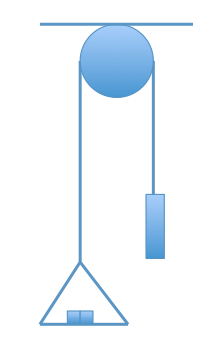If we assumed the pulley had internal friction, how would the initial and final accelerations of the platform with the individual masses change?

Initial acceleration will decrease; final acceleration will increase

Initial acceleration will remain the same; final acceleration will remain the same

Initial acceleration will decrease; final acceleration will remain the same

Initial acceleration will iecrease; final acceleration will remain the same

Initial acceleration will decrease; final acceleration will remain the same

Explanation:

When the rope is around the pulley, the acceleration is slower due to internal friction, thus the initial acceleration of the platform would be lower. Once the platform was in free fall, however, the acceleration due to gravity is constant at 9.8 m/s2; thus, the final acceleration of the platform is the same in both scenarios.

### Example Question #2 : Displacement, Velocity, And Acceleration

A runner completes a lap on a circular track in three minutes. The track has a radius of 210ft. The runner ran at a constant speed around the track.

Which of the following statements is true?

The runner's average velocity is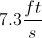The runner's average speed is greater than the runner's average velocity

The runner's total distance traveled is equal to the runner's total displacement

The runner never accelerated while running around the track

The runner's average speed is greater than the runner's average velocity

Explanation:

This is a question that helps you see how displacement and velocity compare to distance and speed, respectively. Remember that the runner starts and stops at the same point on the track, so her total displacement is zero feet.

Since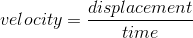, the runner's average velocity is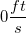. Since the runner's average speed is greater than her average velocity, we can conclude this is the correct answer. Her average speed refers to her distance, rather than displacement, and is greater than zero.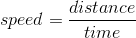This is also important to remember! Since the runner changed direction on the track, she accelerated and decelerated accordingly based on her position on the track. She did not have a constant acceleration.

### Example Question #3 : Displacement, Velocity, And Acceleration

Three blocks (in left-to-right order: block A, block B, block C) are pushed along a frictionless horizontal surface by a 50N force to the right, which acts on block A.

If the masses of the blocks are given by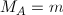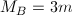, and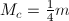, which of the following best describes the relationship between the accelerations of the blocks?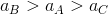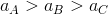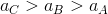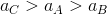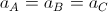The three blocks must remain in contact as they move, so they will each have the same velocity and acceleration regardless of their different masses. So,.